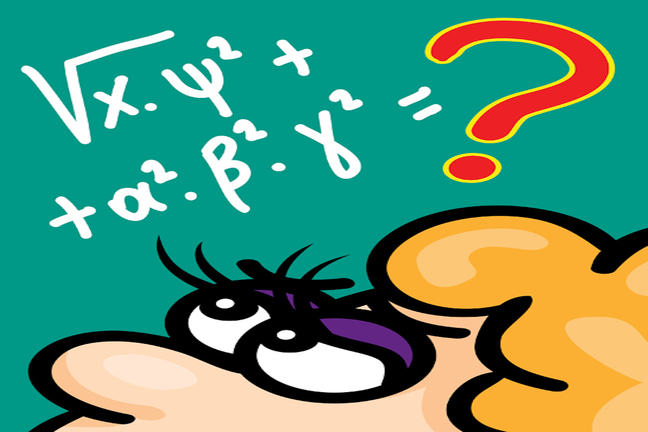## Want to keep learning?

This content is taken from the Weizmann Institute of Science's online course, Maths Puzzles: Cryptarithms, Symbologies and Secret Codes. Join the course to learn more.
3.10

## Weizmann Institute of Science# A bit of history - different ways operators were symbolised through history

Probably the most difficult task a mathematician or a math historian encounters in their day-to-day job is understanding the language. The language of math is symbolic. There are symbols for numbers - we saw that last week; there are symbols for mathematical operations: +, -, x etc. and there are symbols for other mathematical objects such as sets, geometrical shapes and so on. Unfortunately, although math is an international language, there are still today different notations for the same thing, for example, in symmetry, the following five (!) notations all mean the same thing, and are all used today!

xx means the same as pg means the same as C2II means the same as D1gg means the same as W13

This makes things very awkward for people who actually want to try and understand what’s going on! Thank goodness, John H. Conway, Heidi Burgiel and Chaim Goodman-Strauss wrote a dictionary style translation of all the different symmetry notations like the one above at the back of their monumental book, “The Symmetry of Things”.

If you’re a historian, things get even worse. Throughout history, for various reasons, different symbols evolved for the same mathematical objects. Even those symbols that are now internationally (more or less) accepted looked different. This makes it very difficult to decipher things that notable mathematicians like Isaac Newton or Gottfried Wilhelm Leibniz wrote in the past. In fact, both these mathematicians invented calculus at the same time, using different notations! Leibniz used the first letter of the Latin word summa, which at that time was an elongated s to symbolize integration, whereas Newton used different notations at the same time (?!) for integrals, one of them is a small vertical bar above the integrand.

The integral wasn’t the first symbol that Leibniz invented in competition with other symbols for the same thing. In 1698, he wrote: “I do not like X as a symbol for multiplication…” and introduced a dot instead of the usual x. Indeed, dots are still used to indicated multiplication in certain cases, such as scalar multiplication of matrices, and in modern algebra the symbol for multiplication is omitted altogether!

If you are interested in the history of mathematical symbols, here is one of the best explanations on the web.